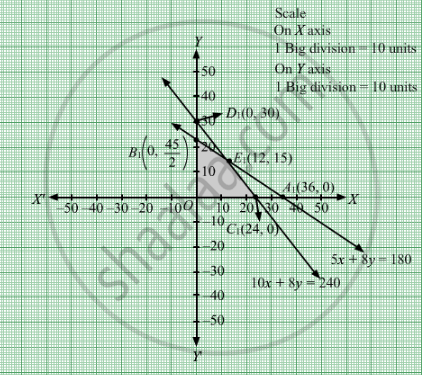# A Company Manufactures Two Types of Toys a and B. Type a Requires 5 Minutes Each for Cutting and 10 Minutes Each for Assembling. - Mathematics

Sum

A company manufactures two types of toys A and B. Type A requires 5 minutes each for cutting and 10 minutes each for assembling. Type B requires 8 minutes each for cutting and 8 minutes each for assembling. There are 3 hours available for cutting and 4 hours available for assembling in a day. The profit is Rs 50 each on type A and Rs 60 each on type B. How many toys of each type should the company manufacture in a day to maximize the profit?

#### Solution

Let toys of type A and toys of type B were manufactured.

The given information can be tabulated as follows:

 Cutting time (minutes) Assembling time (minutes) Toy A(x) 5 10 Toy B(y) 8 8 Availability 180 240

The constraints are

$5x + 8y \leq 180$
$10x + 8y \leq 240$

The profit is Rs 50 each on type A and Rs 60 each on type B. Therefore, profit gained on toys of type A and toys of type is Rs 50x and Rs 60 y respectively.
Total profit  = Z =  $50x + 60y$ The mathematical formulation of the given problem is
Max Z =  $50x + 60y$ subject to

$5x + 8y \leq 180$
$10x + 8y \leq 240$

First we will convert inequations into equations as follows:
5x + 8y = 180, 10x + 8y = 240, x = 0 and y = 0

Region represented by 5x + 8y ≤ 180:
The line 5x + 8y = 180 meets the coordinate axes at A1(36, 0) and $B_1 \left( 0, \frac{45}{2} \right)$ respectively. By joining these points we obtain the line 5x + 8y = 180. Clearly, (0,0) satisfies the 5x + 8y = 180. So, the region which contains the origin represents the solution set of the inequation 5x + 8y ≤ 180.

Region represented by 10x + 8y ≤ 240:
The line 10x + 8y = 240 meets the coordinate axes at C1(24, 0) and $D_1 \left( 0, 30 \right)$  respectively. By joining these points we obtain the line 10x + 8y = 240. Clearly (0,0) satisfies the inequation 10x + 8y ≤ 240. So,the region which contains the origin represents the solution set of the inequation 10x + 8y ≤ 240.

Region represented by ≥ 0 and y ≥ 0:
Since, every point in the first quadrant satisfies these inequations. So, the first quadrant is the region represented by the inequations x ≥ 0, and y ≥ 0.
The feasible region determined by the system of constraints 5x + 8y ≤ 180, 10x + 8y ≤ 240, x ≥ 0 and y ≥ 0 are as follows.
The feasible region is shown in the figureThe corner points are  B$\left( 0, \frac{45}{2} \right)$  E1(12, 15) and C1(24, 0).
The values of Z at the corner points are

 Corner points Z = $50x + 60y$ O 0 B1 1350 E1 1500 C1 1200

The maximum value of Z is 1500 which is at E1(12, 15).

Thus, for maximum profit, 12 units of toy A and 15 units of toy B should be manufactured.
Concept: Graphical Method of Solving Linear Programming Problems
Is there an error in this question or solution?

#### APPEARS IN

RD Sharma Class 12 Maths
Chapter 30 Linear programming
Exercise 30.4 | Q 30 | Page 54